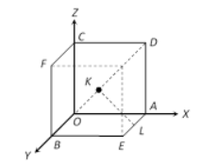Open in App
Not now

# Program to find the shortest distance between diagonal and edge skew of a Cube

• Difficulty Level : Medium
• Last Updated : 17 Mar, 2021

Given an integer A, denoting the length of a cube, the task is to find the shortest distance between the diagonal of a cube and an edge skew to it i.e. KL in the below figure.Examples :

Input: A = 2
Output: 1.4142
Explanation:
Length of KL = A / √2
Length of KL = 2 / 1.41 = 1.4142

Input: A = 3
Output: 2.1213

Approach: The idea to solve the problem is based on the following mathematical formula:

Let’s draw a perpendicular from K to down face of cube as Q.
Using Pythagorean Theorem in triangle QKL,

KL2 = QK2 + QL2

l2 = (a/2)2 + (a/2)2

l2 = 2 * (a/2)2

l = a / sqrt(2)

Below is the implementation of the above approach:

## C++

 `// C++ program for the above approach` `#include ``using` `namespace` `std;` `// Function to find the shortest distance``// between the diagonal of a cube and``// an edge skew to it``float` `diagonalLength(``float` `a)``{``    ``// Stores the required distance``    ``float` `L = a / ``sqrt``(2);` `    ``// Print the required distance``    ``cout << L;``}` `// Driver Code``int` `main()``{``    ``// Given side of the cube``    ``float` `a = 2;` `    ``// Function call to find the shortest``    ``// distance between the diagonal of a``    ``// cube and an edge skew to it``    ``diagonalLength(a);` `    ``return` `0;``}`

## Java

 `// Java program for the above approach` `class` `GFG {` `    ``// Function to find the shortest``    ``// distance between the diagonal of a``    ``// cube and an edge skew to it``    ``static` `void` `diagonalLength(``float` `a)``    ``{``        ``// Stores the required distance``        ``float` `L = a / (``float``)Math.sqrt(``2``);` `        ``// Print the required distance``        ``System.out.println(L);``    ``}` `    ``// Driver Code``    ``public` `static` `void` `main(String[] args)``    ``{``        ``// Given side of the cube``        ``float` `a = ``2``;` `        ``// Function call to find the shortest``        ``// distance between the diagonal of a``        ``// cube and an edge skew to it``        ``diagonalLength(a);``    ``}``}`

## Python3

 `# Python3 program for the above approach` `from` `math ``import` `sqrt` `# Function to find the shortest``# distance between the diagonal of a``# cube and an edge skew to it``def` `diagonalLength(a):``  ` `    ``# Stores the required distance``    ``L ``=` `a ``/` `sqrt(``2``)` `    ``# Print the required distance``    ``print``(L)`  `# Given side of the cube``a ``=` `2` `# Function call to find the shortest``# distance between the diagonal of a``# cube and an edge skew to it``diagonalLength(a)`

## C#

 `// C# program for the above approach` `using` `System;``class` `GFG {` `    ``// Function to find the shortest``    ``// distance between the diagonal of a``    ``// cube and an edge skew to it``    ``static` `void` `diagonalLength(``float` `a)``    ``{``        ``// Stores the required distance``        ``float` `L = a / (``float``)Math.Sqrt(2);` `        ``// Print the required distance``        ``Console.Write(L);``    ``}` `    ``// Driver Code``    ``public` `static` `void` `Main()``    ``{``        ``// Given side of the cube``        ``float` `a = 2;` `        ``// Function call to find the shortest``        ``// distance between the diagonal of a``        ``// cube and an edge skew to it``        ``diagonalLength(a);``    ``}``}`

## PHP

 ``

## Javascript

 ``

Output:

`1.41421`

Time Complexity: O(1)
Auxiliary Space: O(1)

My Personal Notes arrow_drop_up